Welcome to part-4 of the series. Let’s start with where we left.

Question 16- Explain the concept of hoisting in JavaScript?Answer-Hosting means we can use a variable even before declaring it. When the compiler runs and finds all the var declaration, it sorts of move them to the top of the file.

Lets consider this simple example. Here we are doing operations on a , b and c without declaring them first. They are declared on line 5, 6, 7 and yet the interpretor doesn’t throws a runtime error. It is because it doesn’t matter where the variables are declared. They are always hoisted to the top by when the compiler makes the first pass.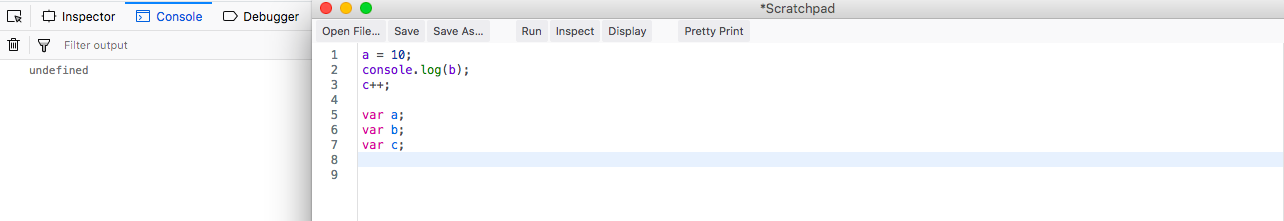hoisting explained

This is also true for function declaration, as the compiler also treats function declaration as variable declaration as in JS all function declaration are object declaration.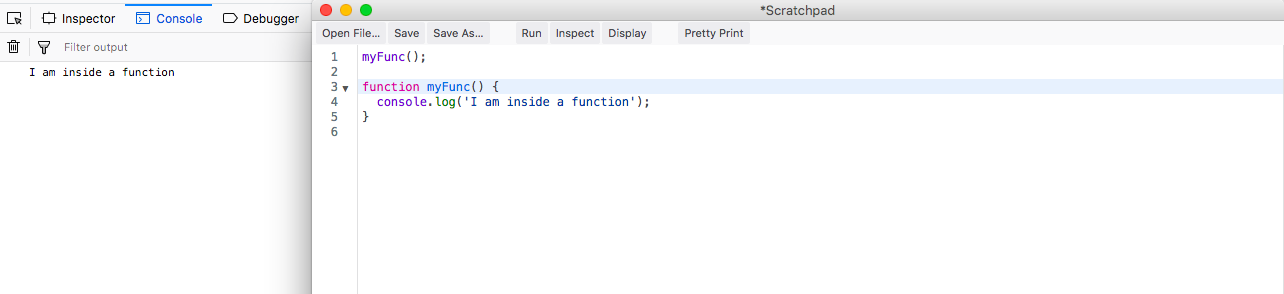function declaration

But the same is not true about function expressions. Let’s see the below example.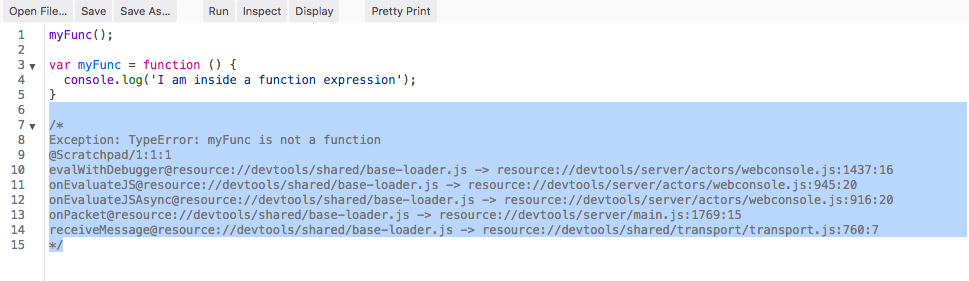function expressions

Also cannot use let variables before declaring them. It will give reference error.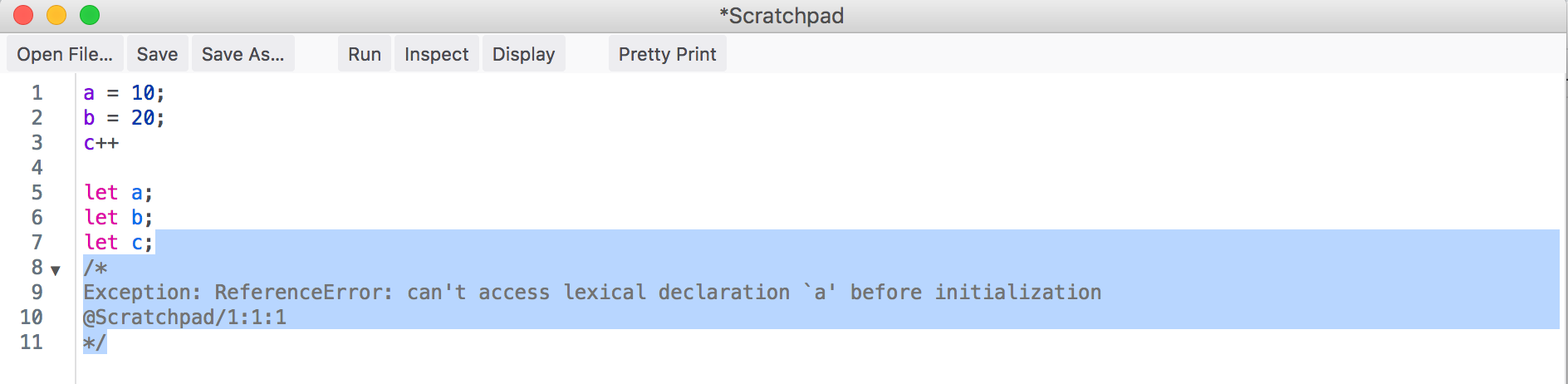let also gives error

Question 17- What is event bubbling and capturing in JavaScript?Answer-Event bubbling means when an event occurs in an webpage on an element, it bubbles to the top. Meaning the parent to the parent and all the way to the body.

Event capturing is the opposite of bubbling and it means when you click on parent element, it goes till the child.

Note that Event bubbling is the default behavior of a browser and event capturing have to be enabled.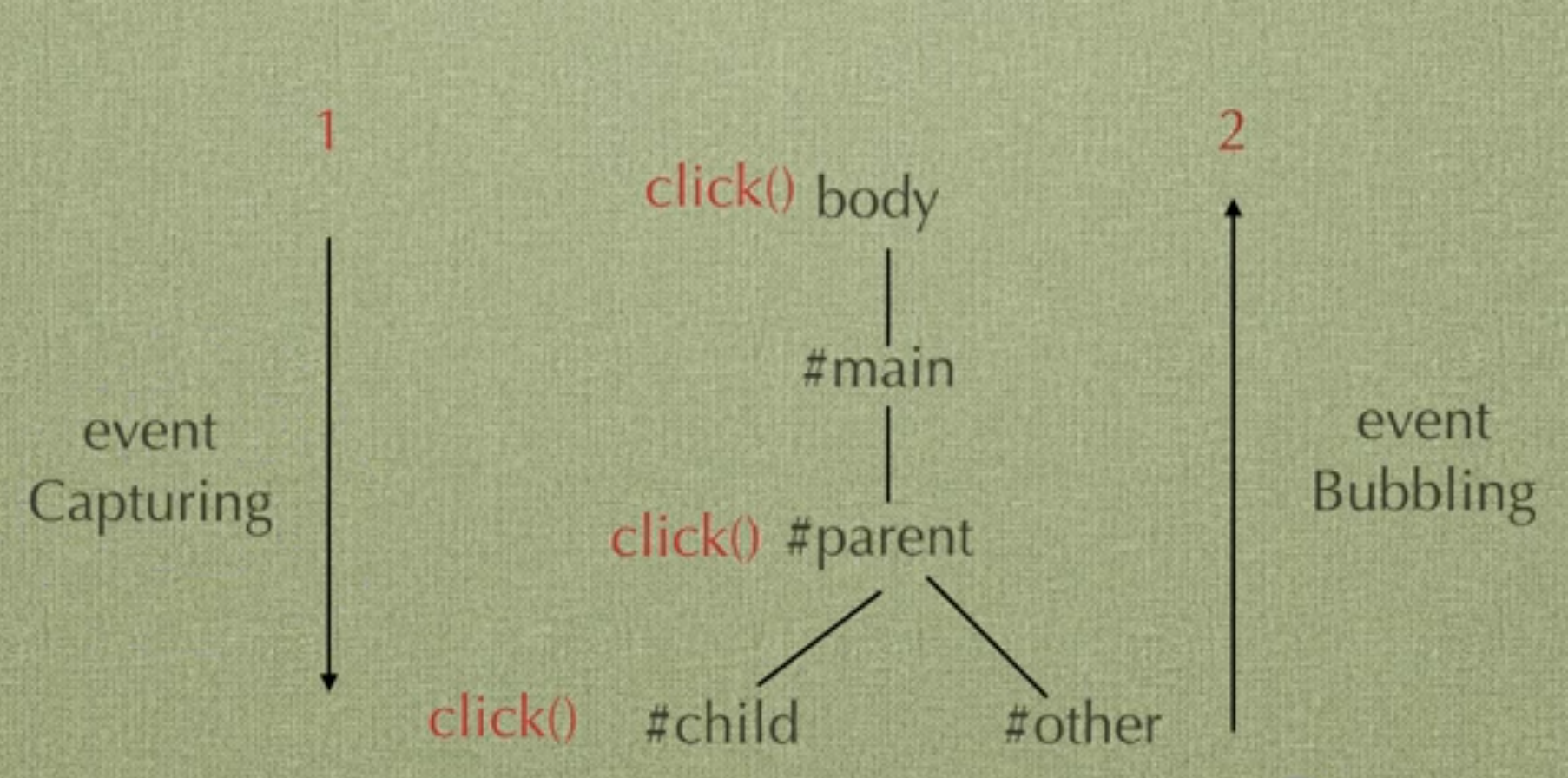event bubbling and capturing

Let see both through an example. The jsfiddle to the same is here.

We will first see Event Bubbling. The HTML contains an parent element containing a child element called Child1. When we click on the button, first the console log of child is displayed and then the parent is displayed.Event bubbling

We will now see Event Capturing. The HTML contains an parent2 element containing a child element called Child2. When we click on the button, first the console log of parent is displayed and then the child is displayed. Notice that in addEventListener we have to pass a value of true.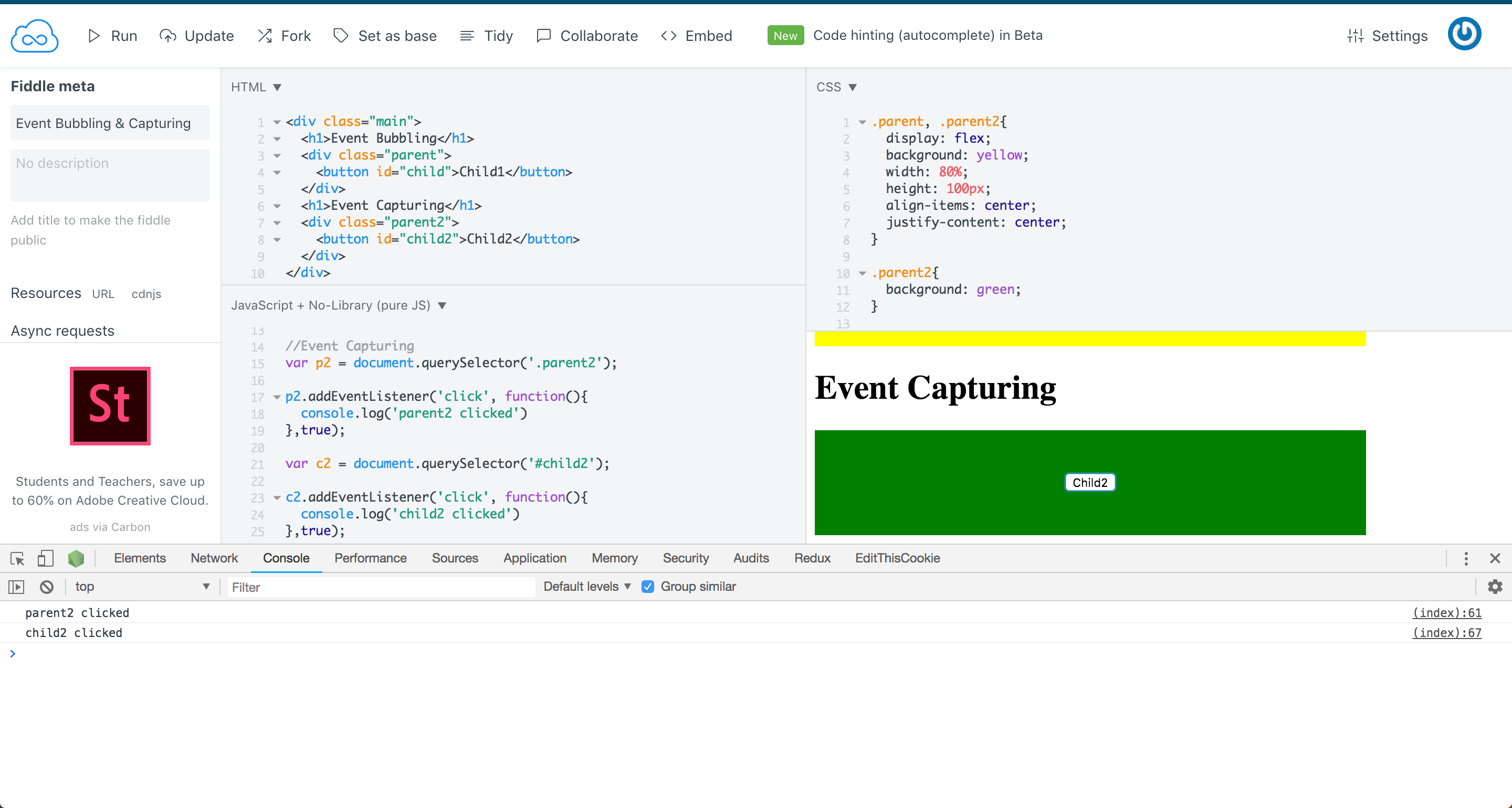Event Capturing

Question 18- What is IIFE(Immediately Invoked Function Expression)?Answer-IIFE means function expressions which are defined and invoked at the same time.

Let consider the below example. As you can see IIFE is nothing but a function expression, but it is defined and invoked at the same time.

``````//Function expression
let funcE = function(x) {
return x + 1;
}

funcE(2); //Outputs - 3

//IIFE
(function(x){
return x + 1;
})(4);

//Outputs - 5
``````

Question 19- What are promises in JavaScript?Answer-Promises in JavaScript are like promises in real life. You promise to do something, and then it is done or not done. In JavaScript a Promise either sent a resolve or reject. Then the promise is captured and if resolve is sent, the statements inside “then” are executed. But if reject is sent, the statements inside “catch” are executed.

Consider the below example. Here inside promiseCleanRoom we generate a random number between 0 and 1. If the number is greater the 0.5, we send a resolve or else we send a reject. Depending on the case either .then is executed or .catch is executed.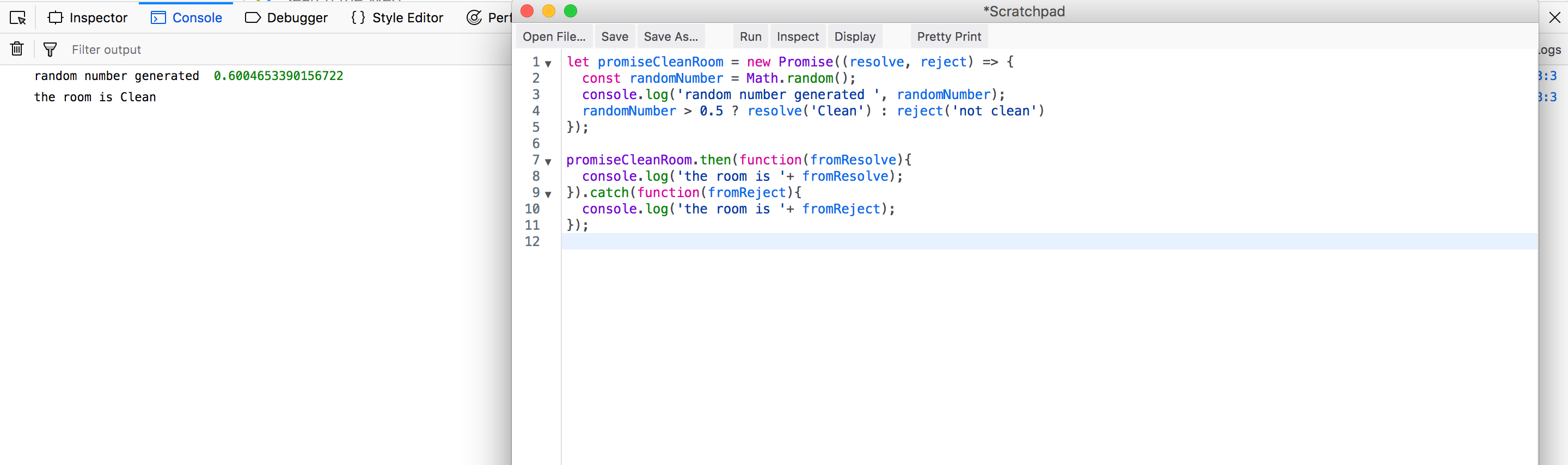resolve case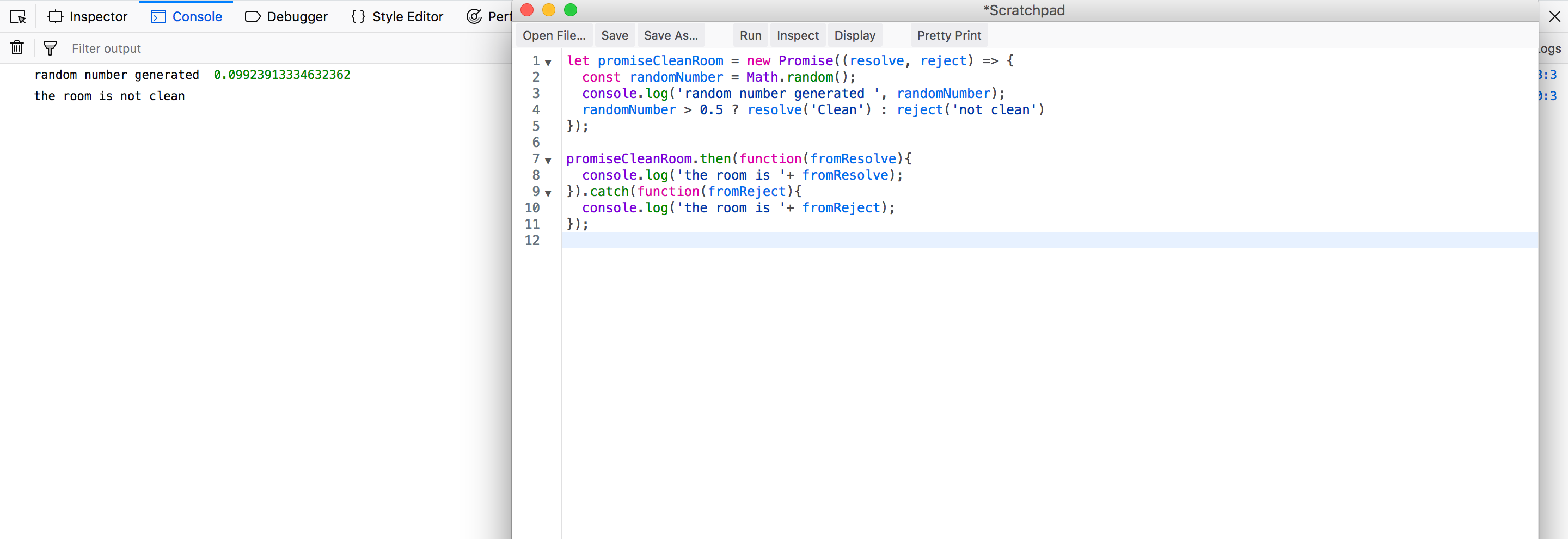reject case

Promises are used a lot to do the API calls in REST endpoints. Fetch function or libraries like axios returns a promise resolve or reject and then we need to have an .then and .catch block for the action.

Question 20- Explain nested promises in JavaScript?Answer-Nested promises are set of promises in which , the result of one promise we call another in the .then statement. It is very useful in practical applications, where the result of fetch from an API endpoint will result in sending the data to another endpoint. Nested promises can also be done with callback functions, but the code get complicated soon.

Let’s look at an example of nested promises. Here we have three functions which return promises- cleanRoom, removeGarbage and winIcecream. Now only the cleanRoom is returning resolve() or reject() depending on random number. The other two functions are returning only resolve() for simplicity sake.

Now, when the cleanRoom function is run and return a resolve(), then the immediate .then block will be run or else we will go to the .catch block. In the .then we are returning the next removeGarbage and in it’s .then we are returning the winIcecream. We are passing the message from one function to other, so it will be appended.

``````let cleanRoom = function() {
return new Promise(function(resolve, reject) {
const randomNumber = Math.random();
console.log('random number generated ', randomNumber);
randomNumber > 0.5 ? resolve('Cleaned the room,') : reject('Too lazy to clean')

});
};

let removeGarbage = function(message) {
return new Promise(function(resolve, reject) {
resolve(message + ' removed Garbage,');
});
};

let winIcecream = function(message) {
return new Promise(function(resolve, reject) {
resolve( message + ' won Icecream');
});
};

cleanRoom().then(function(result){
return removeGarbage(result);
}).then(function(result){
return winIcecream(result);
}).then(function(result){
console.log('All Finished - ' + result);
}).catch(function(res){
console.log('Nothing Done, you are ' + res);
})
``````

On running the code and getting a resolve, because we random number greater then 0.5, we get below output.

``````random number generated  0.5762584054959189
All Finished - Cleaned the room, removed Garbage, won Icecream
``````

On running the code and getting a reject, because we random number less then 0.5, we get below output.

``````random number generated  0.16816251264259796
Nothing Done, you are Too lazy to clean
``````

Question 21- Explain callback functions in JavaScript?Answer-In JavaScript you can pass functions to other function also as arguments and execute at a later time. These are know as callback functions.

Let’s look at the example below. Here we call function y with function x as argument and then from inside y, calling it after some console log.

``````let x = function() {
console.log('I am called from inside a function');
}

let y = function(callBack) {
console.log('I am main function');
callBack();
}

y(x);
//Output -
//I am main function
//I am called from inside a function
``````

When we use anonymous function inside our function call for callback like in the below code.

``````//Callback as anonymous function
let calc = function(num1, num2, callback) {
return callback(num1, num2);
}

console.log(calc(4, 2, function(a, b){
return a - b;
}));
//Output - 2
``````

This type of code is actually used in implementation of map, reduce and filter in JavaScript.

Question 22- Implement your version of Array methods forEach, map and filter in vanilla JavaScript?Answer-We can implement the Array methods by using callbacks and that is how it is implemented in JavaScript engine.

forEach We are implementing our version by having the callback attached to the prototype, which is available to every JS function. Here “this” is equal to the array. So, we are iteration over it using traditional for loop and calling the callback function on each iteration.

``````Array.prototype.myEach = function(callback) {
for (var i = 0; i < this.length; i++)
callback(this[i], i, this);
};

//tests
var arr = ['Software Dev', 'Web Dev', 'FrontEnd Dev', 'BackEnd Dev'];

arr.myEach(function(word) {
console.log(word);
});

//Output-
//Software Dev
//Web Dev
//FrontEnd Dev
//BackEnd Dev
``````

map We are implementing map, by creating an empty array in our function and then pushing it in the iteration. From inside the push we are calling our callback, so when we are calling it we can use something like Math.sqrt to change an individual item.

``````Array.prototype.myMap = function(callback) {
arr = [];
for (var i = 0; i < this.length; i++)
arr.push(callback(this[i], i, this));
return arr;
};

//tests
var arrs = ['Software Dev', 'Web Dev', 'FrontEnd Dev'];
var numbers2 = [1, 4, 9];

var goodT = arrs.myMap(function(n) {
return n;
});

var squareRoot = numbers2.myMap(function(num) {
return Math.sqrt(num);
});

console.log(goodT); // [ 'Software Dev', 'Web Dev', 'FrontEnd Dev' ]
console.log(squareRoot); // [ 1, 2, 3 ]
``````

filter We are implementing map also, by the same process as forEach and filter. But as we know that filter goes through the array and gives an subset of elements depending on boolean expression. Here when we iterate through the array and call the callback in a if statement, and depending on it’s passing push it to the array.

``````Array.prototype.myFilter = function(callback) {
arr = [];
for (var i = 0; i < this.length; i++) {
if (callback(this[i], i, this))
arr.push(this[i]);
}
return arr;
};

//tests
var numbers = [1, 20, 30, 80, 2, 9, 3];
var newNum = numbers.myFilter(function(n) {
return n <= 10;
});
console.log(newNum); // [ 1, 2, 9, 3 ]
``````

Question 23- Implement your version of Array methods some, every and reduce in vanilla JavaScript?Answer-We will be implementing *some, every and reduce *in the same way as we implemented forEach, map and filter.

some The Array prototype method some returns true if any element of the array fulfils the condition we are testing. Here also like filter, we will have the callback been checked in a if statement in every iteration. This will test our condition and even if one of it pass we return a true and get out of loop. If the iteration is complete, it means not a single element satisfy condition and we return false.

``````Array.prototype.mySome = function(callback) {
for (var i = 0; i < this.length; i++) {
if (callback(this[i], i, this))
return true;
}
return false;
};

//tests
var passed = [12, 5, 8, 130, 44].mySome(function(element) {
return (element >= 200);
});
console.log('some: ' + passed); //some: false

var passed = [12, 5, 8, 130, 44].mySome(function(element) {
return (element >= 100);
});
console.log('some: ' + passed); //some: true
``````

every It is the opposite of some. The method “every” will return true only if every element passes the test. Here we do a trick in the if statement. We check the opposite of the test, so say if element should be greater then 10, we check for less then 10. So, if that the case we return false. And if we iterate through whole loop, means all element satisfy the condition so we return true.

``````Array.prototype.myEvery = function(callback) {
for (var i = 0; i < this.length; i++) {
if (!callback(this[i], i, this))
return false;
}
return true;
};

//tests
var passed = [12, 5, 8, 130, 44].myEvery(function(element) {
return (element >= 10);
});
console.log(passed); // false
passed = [12, 54, 18, 130, 44].myEvery(function(element) {
return (element >= 10);
});
console.log(passed); // true
``````

reduce The method reduce is considered the hardest in the Array prototype methods to master. It is because it’s concept is quite different. It takes the whole array and iterate through it and returns a single value. It is useful to do sum/multiply/subtract of all the elements of an array, but is more powerful then that. To do this it have an accumulator, which is used to hold the number in each run. This accumulator will be operated with the current value of the iteration. Now in our implementation, we first check whether an accumulator was supplied or else make it to undefined. Now inside the loop we update the accumulator variable by using the callback.

``````Array.prototype.myReduce = function(callback, initialVal) {
var accumulator = (initialVal === undefined) ? undefined : initialVal;
for (var i = 0; i < this.length; i++) {
if (accumulator !== undefined)
accumulator = callback.call(undefined, accumulator, this[i], i, this);
else
accumulator = this[i];
}
return accumulator;
};

//tests
var numbers3 = [20, 20, 2, 3];
var total = numbers3.myReduce(function(acc, curr) {
return acc + curr;
}, 10);
console.log(total); // 55

total = numbers3.myReduce(function(acc, curr) {
return acc + curr;
});
console.log(total); // 45

let obj = [{x: 1}, {x:2}, {x:3}];
let objTotal = obj.myReduce(function(acc, curr) {
return acc + curr.x;
},0);
console.log(objTotal); //6
``````

Question 24- Implement multiply(2)(3)(4)(10) using currying in vanilla JavaScript?Answer-Named after Haskell Brooks Curry, currying is the process of breaking down a function into a series of function that take a single argument.

Here is a simple example to write a simple multiply function and then the function with currying.

``````//Normal multiply function
function mult(a, b, c, d) {
return a * b * c * d;
}

//Currying multiply function
function multiply(a) {
return (b) => {
return (c) => {
return (d) => {
return a * b * c * d;
}
}
}
}

console.log(mult(2, 3, 4 , 6));  //144
console.log(**multiply(2)(3)(4)(10)**); //240
``````

Currying works in JavaScript because of closures.

Question 25- Explain recursion in JavaScript?Answer-Recursion is simply when a function calls itself.

Let consider the below simple example to add numbers in a series. It will add any number till 0. Say we pass 3, then 3+2+1 = 6.

``````function add(n) {
if(n<=0)
return 0;
else
}

``````

Now, in recursive function there are two parts. One is termination condition and other is the recursion itself. In the above example If(n≤0) is the termination condition and return n + add(n-1); is the recursion. The termination condition is very important or else the recursion never stops and goes into infinite loop.

The recursion works like below. It works like loop, so the first call will go to recursion part and give “3 + add(2)”. Now the add(2) will be called and will be expended into “2 + add(1)”. After that add(1) will be called and expanded into “1 + add(0)”. Finally the add(0) will trigger the termination condition *If(n≤0) *and produce 0. After this everything will be added 3 + 2 + 1 + 0 to give 6.

``````add(3) => 3 + add(2)
3 + 2 + 1 + add(0)
3 + 2 + 1 + 0
6
``````

Question 26- Explain Higher Order Function in JavaScript?Answer-A Higher Order Function is a function that takes one or more function as argument. We use them at many places in JavaScript, and are used mainly as callback functions.

Consider, the simple JS code that adds an event listener to a button. Here, we have a callback function proveIt.

``````<button id="clicker">So Clickable</button>

var proveIt = function() {
};

``````

These callbacks are usually defined inline as anonymous function. So, the refactored code is below.

``````<button id="clicker">So Clickable</button>

});
``````

The array methods map(), filter(), reduce(), forEach() are also Higher Order Functions.

``````const people = [
{ name: ‘John Doe’, age: 16 },
{ name: ‘Thomas Calls’, age: 19 },
{ name: ‘Liam Smith’, age: 20 },
{ name: ‘Jessy Pinkman’, age: 18 },
];

const peopleAbove18 = people.filter(person => person.age>=18);
``````

This concludes part-4 of Basic JavaScript Interview questions. This is the final part of Basic JavaScript.

This post is also available on DEV.